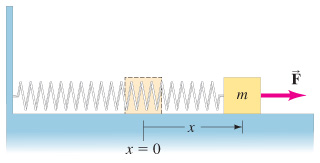# Problem: A mass m is attached to a spring which is held stretched a distance exttip{x}{x} by a force F and then released. The spring compresses, pulling the mass. Assuming there is no friction, determine the speed of the mass m when the spring returns to its normal length ( x = 0 ).Determine the speed of the mass m when the spring returns to half its original extension (x/2).

99% (50 ratings)
###### Problem Details

A mass m is attached to a spring which is held stretched a distance by a force F and then released. The spring compresses, pulling the mass.Assuming there is no friction, determine the speed of the mass m when the spring returns to its normal length ( x = 0 ).

Determine the speed of the mass m when the spring returns to half its original extension (x/2).